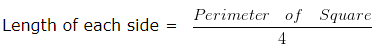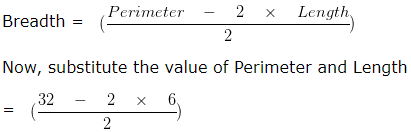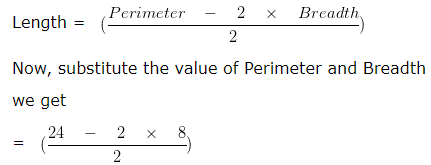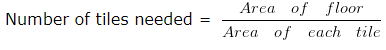# Perimeter Area and Volume Worksheets for Class 5Q.1) Find the area of the Rectangle whose length and breadth are 4 cm and 3 cm respectively.
a) 18 sq. cm
b) 12 sq. cm
c) 20 sq. cm

Q.2) Find the Area of Square whose side is 10 cm ?
a) 90 cm²
b) 100 cm²
c) 96 cm²

Q.3) Find the Perimeter of the Rectangle whose sides are L = 16 cm and B = 12 cm ?
a) 48 cm
b) 56 cm
c) 52 cm

Q.4) Find the perimeter of the Square each of whose side is 12 cm ?
a) 44 cm
b) 48 cm
c) 42 cm

Q.5) Find the side of the Square , whose Perimeter is 38 cm ?
a) 9.5 cm
b) 11.5 cm
c) 6.5 cm

Q.6) If Perimeter of a Rectangle is 32 cm and its length is 6 cm, find its breadth?
a) 10 cm
b) 16 cm
c) 6 cm

Q.7) Find the cost of fencing a square field with sides 16 metres at the rate of ₹ 10 per metre ?
a) ₹ 610
b) ₹ 630
c) ₹ 640

Q.8) What will be the cost of tiling a floor with side 8 m and 10 m if the cost of tiling is Rs. 6 per sq. m ?
a) Rs. 520
b) Rs. 480
c) Rs. 540

Q.9) If the perimeter of a Rectangle is 24 cm and its breadth is 8 cm , find its length ?
a) 4 cm
b) 9 cm
c) 12 cm

Q.10) How many tiles of size 3 m and 4 m can cover the area of whole floor of size 12 m 16 m ?
a) 26
b) 21
c) 16

Q.11) Find the Volume of ice cube if its each side is 5 cm ?
a) 125 cubic cm
b) 128 cubic cm
c) 25 cubic cm

Q.12) Find the Volume of the cuboid if its L = 2 cm , B = 4 cm , H = 5 cm ?
a) 50 cubic cm
b) 40 cubic cm
c) 70 cubic cm

Q.13) A box has length 10 m , breadth 5 m and height 8 m . Find the Volume of box ?
a) 400 cubic m
b) 403 cubic m
c) 398 cubic m

### Perimeter Area and Volume Worksheets for Class 5 Explanations

Q.1) Explanation –

Length of the Rectangle = 4 cm
Breadth of the Rectangle = 3 cm
We know that,
Area of Rectangle = ( Length x Breadth ) sq. unit
= ( 4 x 3 ) sq. cm
= 12 sq. cm
Hence, the Area of Rectangle = 12 sq. cm

Correct Answer – b) 12 sq. cm

Q.2) Explanation – Perimeter Area and Volume Worksheets for Class 5

Given :
Side of Square = 10 cm
Area of Square = ( Side x side ) unit²
= ( 10 x 10 ) cm²
= 100 cm²
Hence, Area of Square = 100 cm²

Correct Answer – b) 100 cm²

Q.3) Explanation –

Rectangle is a four sided polygon having equal opposite sides
Given:
Length = 16 cm
Perimeter of the Rectangle = 2 x ( Length + Breadth ) unit
= 2 x ( 16 + 12 ) cm
= ( 2 x 28 ) cm
= 56 cm
So, Perimeter of the Rectangle is 56 cm

Correct Answer – b) 56 cm

Q.4) Explanation – Perimeter Area and Volume Worksheets for Class 5

Perimeter is a path that surrounds a two-dimensional shape.
We know that all the sides of a Square are equal
Therefore,
Perimeter of Square = ( 4 x Length of each side ) unit
Side of Square = 12 cm
= ( 4 x 12 ) cm
= 48 cm
Hence, Perimeter of Square = 48 cm

Correct Answer – b) 48 cm

Q.5) Explanation –

Perimeter of Square = 38 cm
Square is a four sided polygon having all four sides equal in length.
Therefore,
Perimeter of Square = ( 4 x Length of each side )= 38/4
= 9.5 cm

Correct Answer – a) 9.5 cm

Q.6) Explanation – Perimeter Area and Volume Worksheets for Class 5

Perimeter of Rectangle = 32 cm
Length of Rectangle = 6 cm
As we know that Perimeter of the Rectangle = 2 x ( Length + Breadth )
Perimeter = 2 x Length + 2 x Breadth
2 x Breadth = Perimeter – 2 x Length= (32 – 12)/2
= 20/2
= 10 cm
Breadth of Rectangle = 10 cm

Correct Answer – a) 10 cm

Q.7) Explanation –

Side of square field = 16 metres
To find the cost of fencing first find Perimeter of the field
Perimeter of field = ( 4 x side ) unit
= 4 x 16 metres
= 64 metres
Now,
Cost of fencing square field = Perimeter of field x cost per metre
= 64 x 10
= ₹ 640

Correct Answer – c) ₹ 640

Q.8) Explanation – Perimeter Area and Volume Worksheets for Class 5

It is given that sides of the floor are 8 m and 10 m
Let Length = 8 m
To find the cost of floor , we need to find the area of the floor. As length and breadth of the floor are given
Then,
Area of floor = ( Length x Breadth ) sq. m
= ( 8 x 10 ) sq. m
= 80 sq. m
Cost of 1 sq. m = Rs. 6
Cost of 80 sq. m = Rs. 6 x 80
= Rs. 480

Correct Answer – b) Rs. 480

Q.9) Explanation –

Given :
Perimeter of Rectangle = 24 cm
Breadth of Rectangle = 8 cm
As we know that Perimeter of the Rectangle = 2 x ( Length + Breadth ) unit
Perimeter = 2 x Length + 2 x Breadth
2 x Length = Perimeter – 2 x Breadth= (24 – 16)/2
= 8/2
= 4 cm
Length of Rectangle = 4 cm

Correct Answer – a) 4 cm

Q.10) Explanation – Perimeter Area and Volume Worksheets for Class 5

Area of floor = ( Length x Breadth ) sq. unit
Area of floor = ( 12 x 16 ) sq. m
= 192 sq. m
Area of each tile = ( Length x Breadth ) sq. unit
= ( 3 x 4 ) sq. m
= 12 sq. m= 192/12
= 16 tiles

Q.11) Explanation –

Cube is a 3D shape in which all the sides are equal
Volume of cube = ( side x side x side ) cubic unit
Volume of cube = ( 5 x 5 x 5 ) cubic cm
= 125 cubic cm

Correct Answer – a) 125 cubic cm

Q.12) Explanation – Perimeter Area and Volume Worksheets for Class 5

Length of the cuboid = 2 cm
Breadth of the cuboid = 4 cm
Height of the cuboid = 5 cm
Volume of Cuboid = Length x Breadth x Height
= 2 cm x 4 cm x 5 cm
= 40 cubic cm

Correct Answer – b)40 cubic cm

Q.13) Explanation –

Length of box = 10 m
Breadth of box = 5 m
Height of box = 8 m
It is clear that box is in cubiodal shape
Volume of Cuboid = ( Length x Breadth x Height ) cubic unit
= ( 10 x 5 x 8 ) cubic m
= 400 cubic m

Correct Answer – a) 400 cubic m